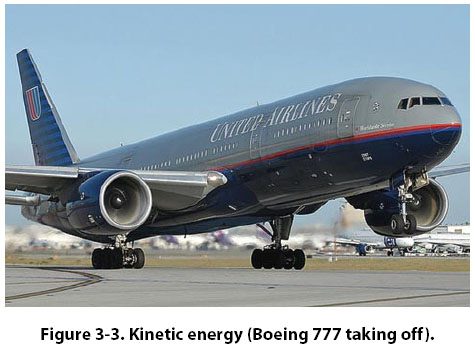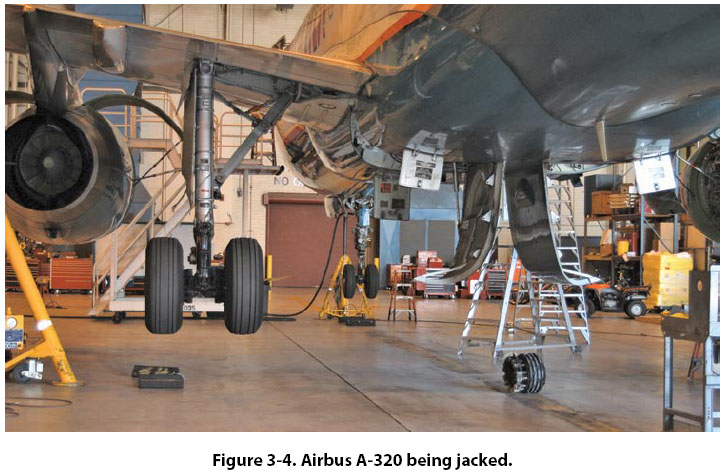Kinetic Energy Kinetic energy is defined as being energy in motion. An airplane rolling down the runway or a rotating flywheel on an engine are both examples of kinetic energy. Kinetic energy has the same units as potential energy, namely foot-pounds or inch-pounds. To calculate the kinetic energy for something in motion, the following formula is used: Kinetic Energy = 1/2 Mass × Velocity2 To use the formula, we will show the mass as weight ÷ gravity and the velocity of the object will be in feet per second. This is necessary to end up with units in foot-pounds. Example: A Boeing 777 weighing 600,000 lb is moving down the runway on its takeoff roll with a velocity of 200 fps. How many foot-pounds of kinetic energy does the airplane possess? [Figure 3-3] Kinetic Energy = 1/2 Mass × Velocity2 Kinetic Energy = 1/2 × 600,000 ÷ 32.2 × 2002 KE = 372,670,000 ft-lbForce, Work, Power, and Torque Force Before the concept of work, power, or torque can be discussed, we must understand what force means. According to the dictionary, force is the intensity of an impetus, or the intensity of an input. For example, if we apply a force to an object, the tendency will be for the object to move. Another way to look at it is that for work, power, or torque to exist, there has to be a force that initiates the process. The unit for force in the English system of measurement is pounds, and in the metric system it is newtons. One pound of force is equal to 4.448 newtons. When we calculate the thrust of a turbine engine, we use the formula “Force = Mass × Acceleration," and the thrust of the engine is expressed in pounds. The GE90-115 turbofan engine (powerplant for the Boeing 777-300), for example, has 115,000 pounds of thrust. Work The study of machines, both simple and complex, is in one sense a study of the energy of mechanical work. This is true because all machines transfer input energy, or the work done on the machine, to output energy, or the work done by the machine. Work, in the mechanical sense of the term, is done when a resistance is overcome by a force acting through a measurable distance. Two factors are involved: (1) force and (2) movement through a distance. As an example, suppose a small aircraft is stuck in the snow. Two men push against it for a period of time, but the aircraft does not move. According to the technical definition, no work was done in pushing against the aircraft. By definition, work is accomplished only when an object is displaced some distance against a resistive force. To calculate work, the following formula is used: Work = Force (F) × distance (d) In the English system, the force will be identified in pounds and the distance either in feet or inches, so the units will be foot-pounds or inch-pounds. Notice these are the same units that were used for potential and kinetic energy. In the metric system, the force is identified in newtons (N) and the distance in meters, with the resultant units being joules. One pound of force is equal to 4.448 N and one meter is equal to 3.28 feet. One joule is equal to 1.36 ft-lb. Example: How much work is accomplished by jacking a 150,000-lb Airbus A-320 airplane a vertical height of 3 ft? [Figure 3-4] Work = Force × Distance = 150,000 lb × 4 ft = 600,000 ft-lbExample: How much work is accomplished when a tow tractor is hooked up to a tow bar and a Boeing 737-800 airplane weighing 130,000 lb is pushed 80 ft into the hangar? The force on the tow bar is 5,000 lb. Work = Force × Distance = 5,000 lb × 80 ft = 400,000 ft-lb In this last example, notice the force does not equal the weight of the airplane. This is because the airplane is being moved horizontally and not lifted vertically. In virtually all cases, it takes less work to move something horizontally than it does to lift it vertically. Most people can push their car a short distance if it runs out of gas, but they cannot get under their car and lift it off the ground.
 ©AvStop Online Magazine                                                                                                                                                      Contact Us              Return To Books